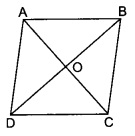# Prove that the sum of the squares of the sides of a rhombus is equal to the sum of the squares of its diagonals.

To do:

We have to prove that the sum of the squares of the sides of a rhombus is equal to the sum of the squares of its diagonals.

Solution:

Let $ABCD$ be a rhombus in which diagonals $AC$ and $BD$ intersect each other at $O$.We know that,

The two diagonals of a rhombus are perpendicular.

Therefore,

In $\triangle \mathrm{AOB}$,

$\mathrm{AB}^{2}=\mathrm{AO}^{2}+\mathrm{OB}^{2}$

$\mathrm{AB}^{2}=(\frac{1}{2} \mathrm{AC})^{2}+(\frac{1}{2} \mathrm{BD})^{2}$

$\mathrm{AB}^{2}=\frac{1}{4} \mathrm{AC}^{2}+\frac{1}{4} \mathrm{BD}^{2}$

$4 \mathrm{AB}^{2}=\mathrm{AC}^{2}+\mathrm{BD}^{2}$

$\mathrm{AB}^{2}+\mathrm{AB}^{2}+\mathrm{AB}^{2}+\mathrm{AB}^{2}=\mathrm{AC}^{2}+\mathrm{BD}^{2}$

$\mathrm{AB}^{2}+\mathrm{BC}^{2}+\mathrm{CD}^{2}+\mathrm{DA}^2=\mathrm{AC}^{2}+\mathrm{BD}^{2}$

Hence proved.